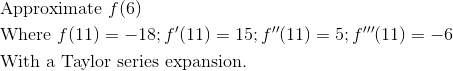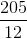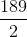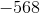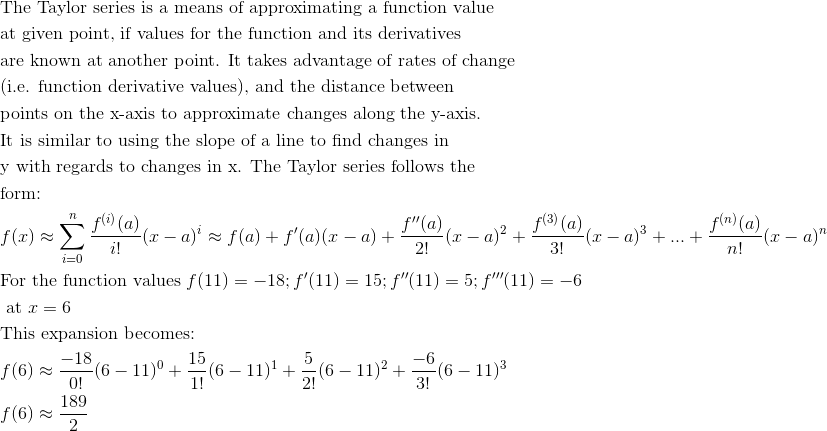AP Calculus BC : Taylor Series

Example Questions

← Previous 1 3 4 5 6 7

Example Question #1 : Ap Calculus Bc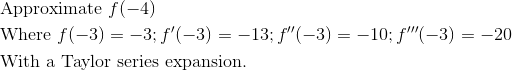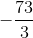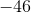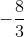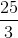Explanation: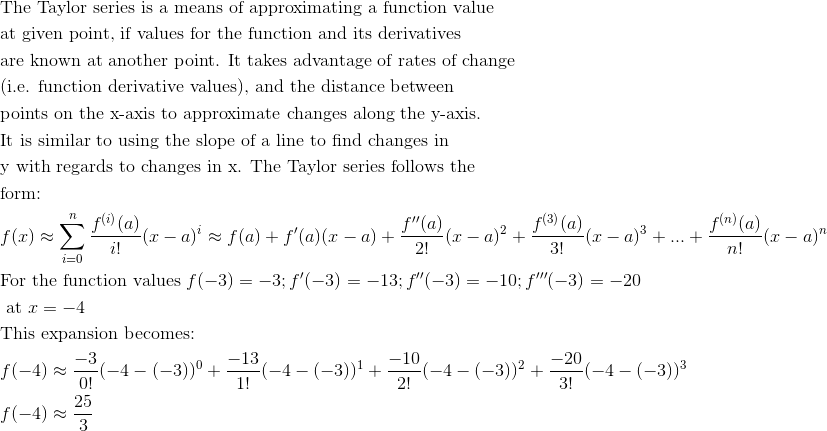Example Question #2 : Ap Calculus Bc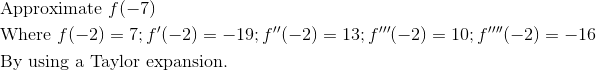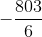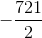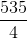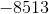Explanation: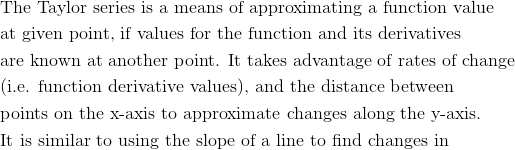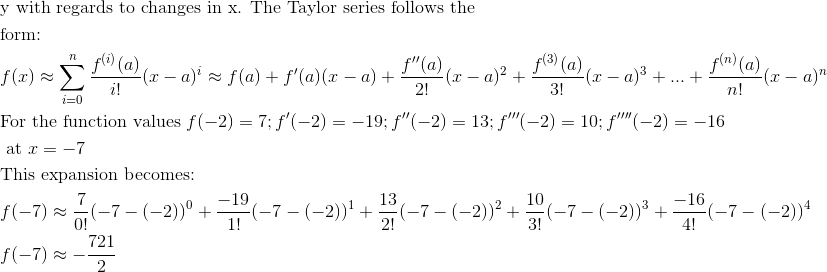Example Question #3 : Ap Calculus Bc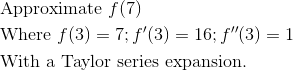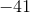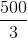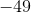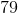Explanation: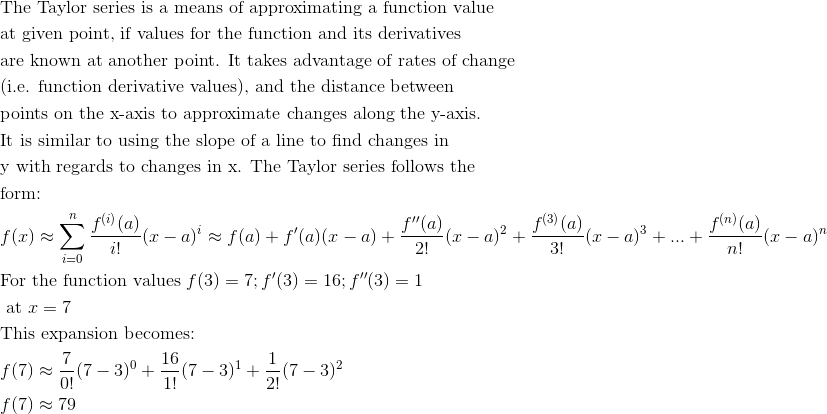Example Question #4 : Ap Calculus Bc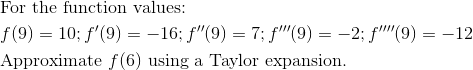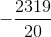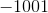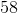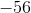Explanation: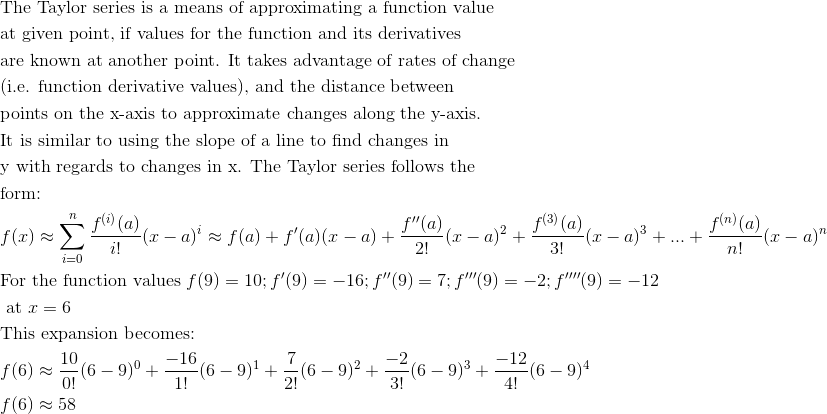Example Question #5 : Ap Calculus Bc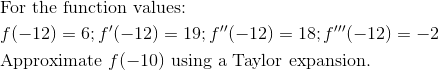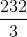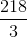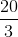Explanation: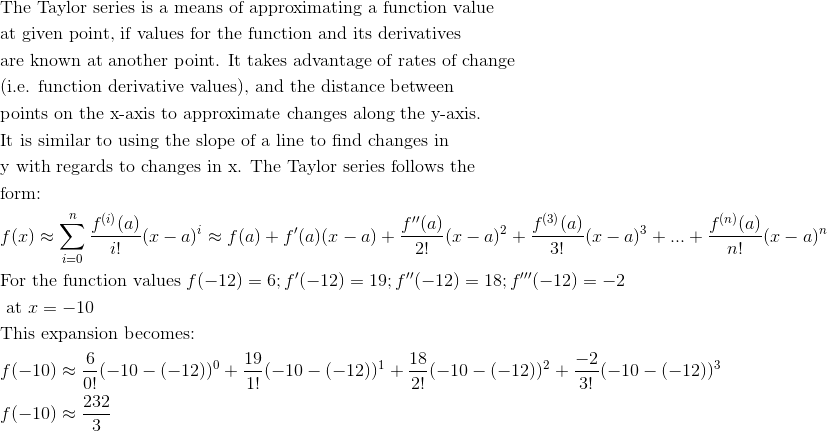Example Question #6 : Ap Calculus Bc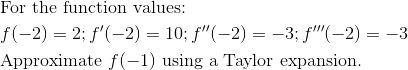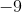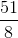Explanation: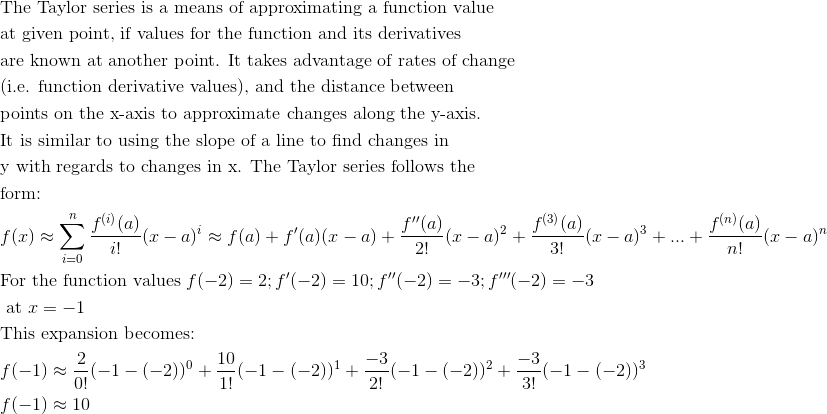Example Question #7 : Ap Calculus Bc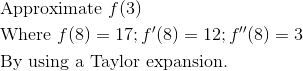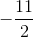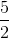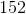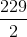Explanation: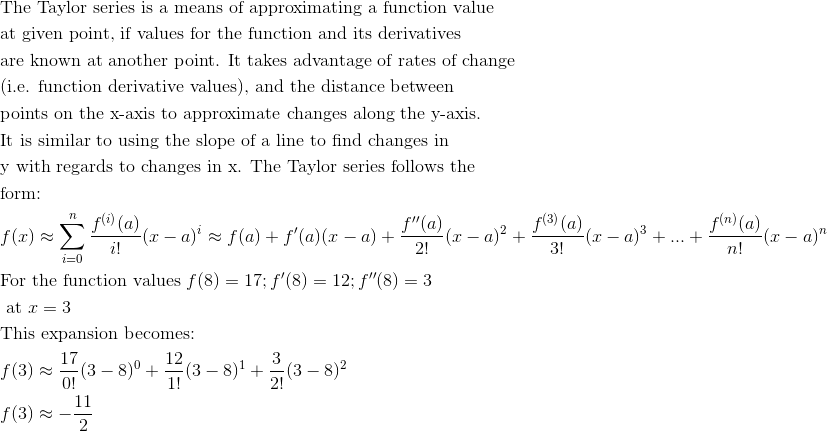Example Question #8 : Ap Calculus Bc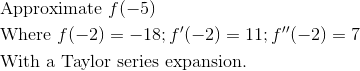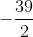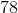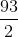Explanation: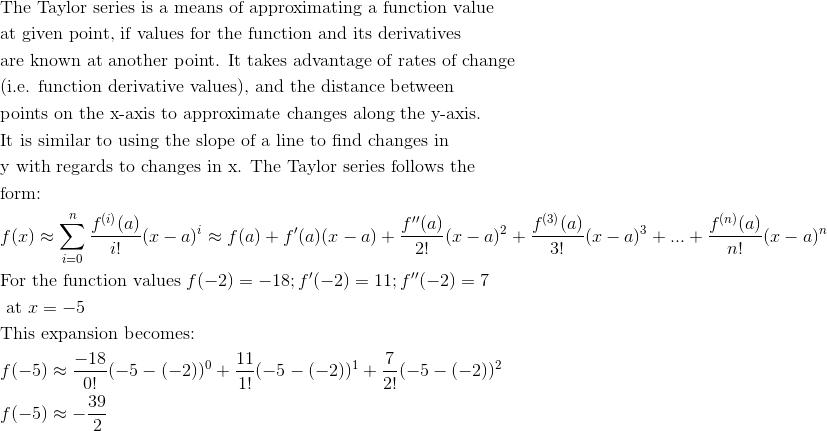Example Question #9 : Ap Calculus Bc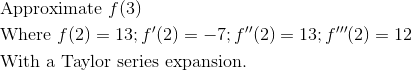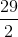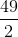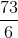Explanation:Example Question #10 : Ap Calculus Bc